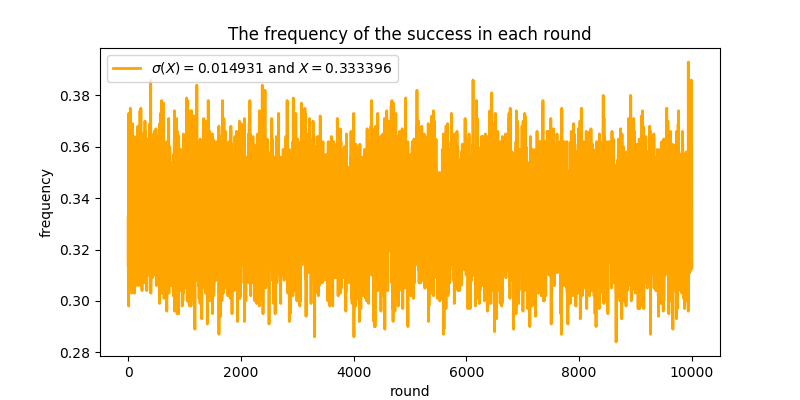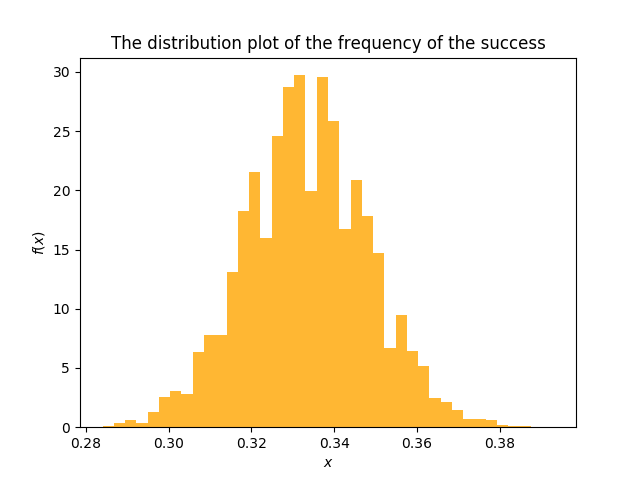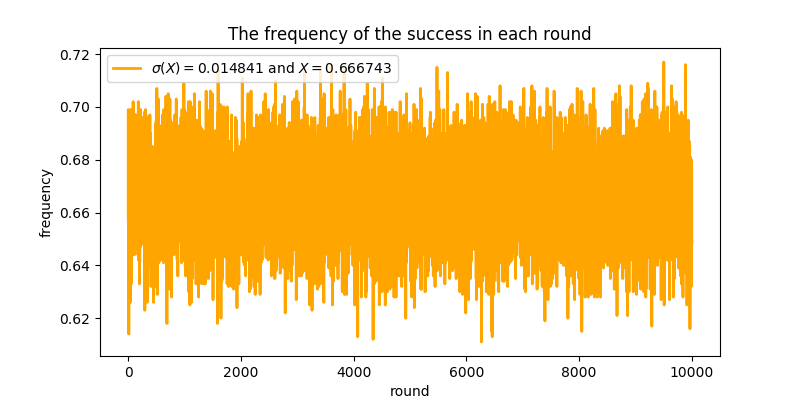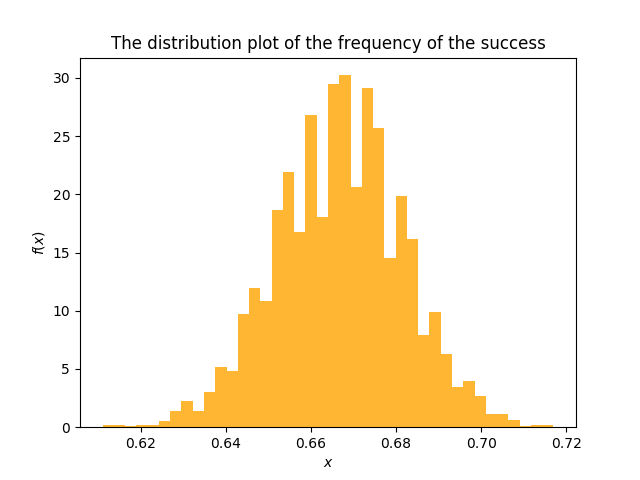## 三门问题的概率表述

### 贝叶斯公式

$$P(A\mid B) = \frac{P(A) P(B\mid A)}{P(B)}.$$

### 三门问题的概率建模

• 事件 $A$：参赛者选择的门后有汽车；
• 事件 $B$：主持人选择的门后有汽车。

$$P(A\mid \bar B) = \frac{P(A) P(\bar B\mid A)}{P(\bar B)}.$$

• 若 $P(A\mid \bar B) < \frac{1}{2}$，则答案是「是」；
• 若 $P(A\mid \bar B) \geqslant \frac{1}{2}$，则答案是「否」。

• 若 $P(\bar B) > \frac{2}{3}$，则 $P(A\mid \bar B) < \frac{1}{2}$，答案是「是」；
• 若 $P(\bar B) \leqslant \frac{2}{3}$，则 $P(A\mid \bar B) \geqslant \frac{1}{2}$，答案是「否」。

## 边缘概率 $P(\bar B)$ 之谜

### 两种答案

• 既然主持人排除了一个错误选项，那么原始问题就变成了二选一的新问题，此时选哪个都一样，中奖概率都是 $\frac{1}{2}$。因此答案是「否」。
• 三扇门的中奖概率都是 $\frac{1}{3}$，参赛者选中的门的中奖概率自然也是 $\frac{1}{3}$；而主持人选择的门打开后，$\frac{1}{3}$ 就「跑到」另一扇门上去了，所以另一扇门的中奖概率是 $\frac{2}{3}$。因此答案是「是」。

### 暗藏的假设

• 主持人并不知道门后的情况，随机选择后恰好门后是羊；特别地，主持人不知道参赛者选择的门后的情况，因而主持人的选择没有带来新的信息量。即：$$P(\bar B) = P(\bar B\mid A)P(A) + P(\bar B\mid\bar A)P(\bar A) = 1\cdot\frac{1}{3} + \frac{1}{2}\cdot\frac{2}{3} = \frac{2}{3}.$$
• 主持人知道门后的情况，因此选择的门后边必然是羊；特别地，主持人知道参赛者选择的门后的情况，因而主持人的选择带来了新的信息量。即：$P(\bar B) = 1$。

## 回到小孩子的思维

• 当参赛者一开始选择汽车时（$\frac{1}{3}$ 概率），不论主持人选择羊A 还是羊B，若参赛者更换选择，都不能赢得汽车
• 当参赛者一开始选择羊A时（$\frac{1}{3}$ 概率），主持人必然选择羊B，若参赛者更换选择，则必然赢得汽车
• 当参赛者一开始选择羊B时（$\frac{1}{3}$ 概率），主持人必然选择羊A，若参赛者更换选择，则必然赢得汽车

## 计算机模拟实验

Python 代码（在此下载）读起来很容易，因此不做详细说明，而只给出简单的解释。对于参赛者的两种选择（在主持人打开一扇门后是否更改选择），代码分别进行了 10000 轮，每轮 1000 次的实验。而后，代码统计每轮 1000 次实验中，参赛者成功赢得汽车的频率；并将 10000 轮频率绘制成图展示。